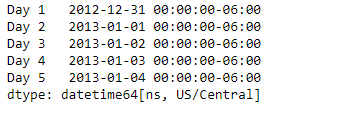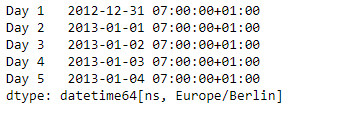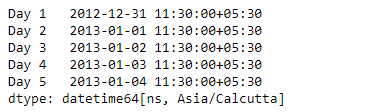# Python | Pandas Series.dt.tz_convert

`Series.dt` can be used to access the values of the series as datetimelike and return several properties. Pandas` Series.dt.tz_convert()` function convert tz-aware Datetime Array/Index from one time zone to another.

Syntax: Series.dt.tz_convert(*args, **kwargs)

Parameter :

tz : Time zone to convert timestamps to.

Returns : same type as self

Example #1: Use `Series.dt.tz_convert()` function to convert the timezone of the timestamps in the given series object.

 `# importing pandas as pd ` `import` `pandas as pd ` ` `  `# Creating the Series ` `sr ``=` `pd.Series(pd.date_range(``'2012-12-31 00:00'``, periods ``=` `5``, freq ``=` `'D'``, ` `                            ``tz ``=` `'US / Central'``)) ` ` `  `# Creating the index ` `idx ``=` `[``'Day 1'``, ``'Day 2'``, ``'Day 3'``, ``'Day 4'``, ``'Day 5'``] ` ` `  `# set the index ` `sr.index ``=` `idx ` ` `  `# Print the series ` `print``(sr) `

Output :Now we will use `Series.dt.tz_convert()` function to convert the timestamps in the given series object to ‘Europe/Berlin’.

 `# convert to 'Europe / Berlin' ` `result ``=` `sr.dt.tz_convert(tz ``=` `'Europe / Berlin'``) ` ` `  `# print the result ` `print``(result) `

Output :As we can see in the output, the `Series.dt.tz_convert()` function has successfully converted the timezone of the timestamps in the given series object to the target timezone.

Example #2 : Use `Series.dt.tz_convert()` function to convert the timezone of the timestamps in the given series object.

 `# importing pandas as pd ` `import` `pandas as pd ` ` `  `# Creating the Series ` `sr ``=` `pd.Series(pd.date_range(``'2012-12-31 00:00'``, periods ``=` `5``, freq ``=` `'D'``, ` `                            ``tz ``=` `'US / Central'``)) ` ` `  `# Creating the index ` `idx ``=` `[``'Day 1'``, ``'Day 2'``, ``'Day 3'``, ``'Day 4'``, ``'Day 5'``] ` ` `  `# set the index ` `sr.index ``=` `idx ` ` `  `# Print the series ` `print``(sr) `

Output :Now we will use `Series.dt.tz_convert()` function to convert the timestamps in the given series object to ‘Asia/Calcutta’.

 `# convert to 'Asia / Calcutta' ` `result ``=` `sr.dt.tz_convert(tz ``=` `'Asia / Calcutta'``) ` ` `  `# print the result ` `print``(result) `

Output :As we can see in the output, the `Series.dt.tz_convert()` function has successfully converted the timezone of the timestamps in the given series object to the target timezone.

My Personal Notes arrow_drop_upCheck out this Author's contributed articles.

If you like GeeksforGeeks and would like to contribute, you can also write an article using contribute.geeksforgeeks.org or mail your article to contribute@geeksforgeeks.org. See your article appearing on the GeeksforGeeks main page and help other Geeks.

Please Improve this article if you find anything incorrect by clicking on the "Improve Article" button below.

Article Tags :

Be the First to upvote.

Please write to us at contribute@geeksforgeeks.org to report any issue with the above content.